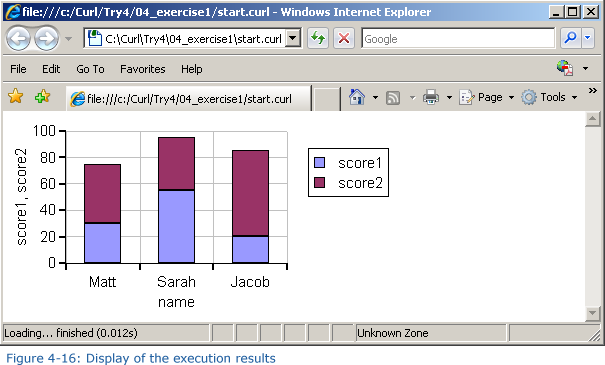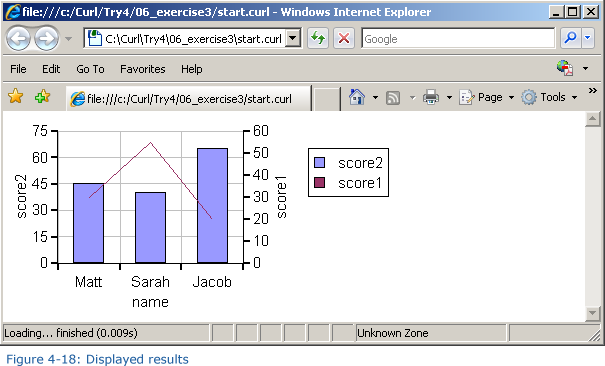Page 8: Extra Practice - Printable Version +- Curl Global Community (https://communities.curl.com) +-- Forum: Tutorials (https://communities.curl.com/forumdisplay.php?fid=3) +--- Forum: Public Training (https://communities.curl.com/forumdisplay.php?fid=4) +---- Forum: Curl IDE Made Easy (https://communities.curl.com/forumdisplay.php?fid=6) +----- Forum: Try 4: Displaying Data as a Graph (https://communities.curl.com/forumdisplay.php?fid=12) +----- Thread: Page 8: Extra Practice (/showthread.php?tid=70) Page 8: Extra Practice - ashimo - 06-20-2011 Extra Practice Let’s use options to change the format and colors of a graph. Practice Problem 1: Basics Define data like that shown below, and then display a cumulative bar graph for the score1 and score2 data fields. For the x-axis label, use the name field. Code:```let data:RecordSet = {RecordSet                          {RecordFields                              {RecordField name, domain = String},                              {RecordField score1, domain = int},                              {RecordField score2, domain = int}                          },                          {RecordData name = Matt, score1 = 30, score2 = 45},                          {RecordData name = Sarah, score1 = 55, score2 = 40},                          {RecordData name = Jacob, score1 = 20, score2 = 65}                      }```Hint: To display the cumulative graph, use stacking-mode = ChartStackingMode.stacked with BarLayer. Solution Solution Program: c:\Curl\Try4\04_exercise1\start.curl Code:```{import * from CURL.GUI.CHARTS} {value     let data:RecordSet =         {RecordSet             {RecordFields                 {RecordField name, domain = String},                 {RecordField score1, domain = int},                 {RecordField score2, domain = int}             },             {RecordData name = Matt, score1 = 30, score2 = 45},             {RecordData name = Sarah, score1 = 55, score2 = 40},             {RecordData name = Jacob, score1 = 20, score2 = 65}         }          let chart:LayeredChart = {LayeredChart                                  height = 5cm,                                  width = 10cm,                                  {BarLayer                                      stacking-mode = ChartStackingMode.stacked,                                      x-axis-data = {ChartDataSeries data, name},                                      {ChartDataSeries data, score1},                                      {ChartDataSeries data, score2}                                  }                              }     chart }``` Practice Problem 2: Basics Display the data used with Practice Problem 1 as a bar graph. In this problem, reverse the directions of the x- and y-axes and then display the graph.Hint: To change the orientation of the graph, use flipped? = true with LayeredChart. Solution Solution Program: c:\Curl\Try4\05_exercise2\start.curl Code:```{import * from CURL.GUI.CHARTS} {value     let data:RecordSet =         {RecordSet             {RecordFields                 {RecordField name, domain = String},                 {RecordField score1, domain = int},                 {RecordField score2, domain = int}             },             {RecordData name = Matt, score1 = 30, score2 = 45},             {RecordData name = Sarah, score1 = 55, score2 = 40},             {RecordData name = Jacob, score1 = 20, score2 = 65}`         }          let chart:LayeredChart = {LayeredChart                                  height = 5cm,                                  width = 10cm,                                  flipped? = true,                                  {BarLayer                                      x-axis-data = {ChartDataSeries data, name},                                      {ChartDataSeries data, score1},                                      {ChartDataSeries data, score2}                                  }                              }     chart }``` Practice Problem 3: Basics Using the same data as that used in Practice Problem 1, display the score1 data as a bar graph, and the score2 data as a line graph, as shown in the figure below:Hint: LayeredChart can have multiple ChartLayers. Solution Solution Program: c:\Curl\Try4\06_exercise3\start.curl Code:```{import * from CURL.GUI.CHARTS} {value     let data:RecordSet =         {RecordSet             {RecordFields                 {RecordField name, domain = String},                 {RecordField score1, domain = int},                 {RecordField score2, domain = int}             },             {RecordData name = Matt, score1 = 30, score2 = 45},             {RecordData name = Sarah, score1 = 55, score2 = 40},             {RecordData name = Jacob, score1 = 20, score2 = 65}         }          let chart:LayeredChart = {LayeredChart                                  height = 5cm,                                  width = 10cm,                                  {BarLayer                                      x-axis-data = {ChartDataSeries data, name},                                      {ChartDataSeries data, score2}                                  },                                  {LineLayer                                      {ChartDataSeries data, score1}                                  }                              }          chart }```